### Wrath from Heaven Part 1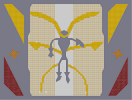Hover over the thumbnail for a full-size version.

Author ghazbaran author:ghazbaran n-art nonplayable not-finished unrated 2007-12-06 2007-12-07 \$Wrath from Heaven Part 1#ghazbaran#none#FJ11111111111LH0000021100FJ11111111511LH0000214000FJ11111402111LH0002140000FJ11113111111LH0011400000FJ11111111111LH11111111111111111111111MQQQQQQI00000000FQQQQQJ00000000000000000000000000000000000000000000000000000000000000000000000000000000000000000000000000000GOLK@00000000000000000?I0IF000000000000000000NOH000GOOLKA000000000?4B11LOKI00II0000000000>EC11MQJOOOLOH00000000000NQI000000IFI00000000000>@000000000000000000000>1A0000000000000000000002D00000000000000000000000000000000000000000000000000000000000000000000000000000000000000000000000000000000LOOOOOOH00000000GOOOOOK1111111111111111111111115000000GK11111111111MI500000GK11112111111MI000000GK11111503111MI000300GK11111111411MI000031GK11111111111MI00000311|0^324,564!0^444,564!0^444,540!0^444,516!0^444,492!0^444,480!0^444,468!0^444,444!0^444,456!0^444,432!0^444,408!0^444,396!0^444,420!0^444,384!0^444,372!0^444,348!0^444,492!0^444,504!0^444,528!0^444,540!0^444,552!0^324,576!0^444,576!0^324,552!0^324,540!0^324,516!0^324,504!0^324,492!0^324,480!0^324,468!0^324,456!0^324,444!0^324,432!0^324,420!0^324,408!0^324,396!0^324,396!0^324,384!0^324,372!0^324,372!0^324,360!0^324,348!0^324,336!0^324,324!0^324,312!0^324,300!0^324,288!0^324,276!0^324,264!0^324,252!0^324,240!0^324,228!0^324,216!0^324,204!0^324,192!0^444,360!0^444,336!0^444,324!0^444,312!0^444,300!0^444,300!0^444,288!0^444,276!0^444,264!0^444,252!0^444,240!0^444,228!0^444,216!0^444,204!0^444,192!0^444,180!0^444,156!0^444,168!0^444,144!0^444,132!0^444,120!0^444,108!0^444,96!0^444,84!0^444,72!0^444,60!0^444,36!0^324,180!0^324,168!0^324,156!0^324,144!0^324,132!0^324,120!0^324,108!0^324,96!0^324,84!0^324,60!0^324,48!0^324,36!0^324,24!0^420,396!0^426,396!0^420,402!0^426,402!0^432,408!0^438,408!0^444,414!0^450,414!0^456,420!0^462,426!0^468,432!0^474,438!0^480,444!0^486,450!0^492,456!0^498,462!0^498,468!0^504,474!0^504,480!0^510,486!0^510,492!0^510,498!0^510,504!0^516,510!0^516,516!0^516,516!0^516,522!0^516,528!0^516,534!0^516,540!0^516,546!0^522,552!0^522,546!0^522,540!0^522,528!0^522,534!0^522,522!0^522,516!0^522,510!0^516,504!0^516,504!0^516,492!0^516,498!0^516,486!0^510,480!0^510,474!0^504,468!0^504,462!0^498,456!0^492,450!0^486,444!0^480,438!0^474,432!0^468,426!0^462,420!0^456,414!0^450,408!0^444,402!0^438,402!0^432,402!0^522,558!0^522,558!0^522,564!0^420,390!0^414,390!0^414,396!0^408,396!0^408,390!0^348,384!0^348,390!0^342,390!0^336,396!0^330,396!0^324,402!0^318,402!0^312,408!0^306,414!0^300,420!0^294,426!0^288,432!0^282,438!0^276,444!0^270,450!0^264,456!0^264,462!0^258,468!0^258,474!0^258,480!0^258,486!0^258,492!0^252,498!0^252,504!0^252,510!0^252,510!0^252,516!0^252,522!0^252,528!0^252,534!0^246,546!0^246,540!0^246,534!0^246,522!0^246,516!0^246,534!0^246,528!0^246,510!0^246,504!0^246,498!0^252,486!0^252,492!0^252,480!0^258,474!0^252,474!0^252,468!0^258,462!0^258,456!0^264,450!0^270,444!0^276,438!0^246,552!0^522,570!0^282,432!0^288,426!0^294,420!0^300,414!0^306,408!0^312,402!0^318,396!0^324,390!0^330,390!0^336,390!0^342,384!0^408,216!0^402,216!0^366,216!0^360,216!0^408,210!0^414,204!0^414,198!0^420,192!0^420,186!0^426,180!0^426,174!0^432,168!0^432,162!0^438,156!0^438,150!0^450,138!0^456,132!0^462,132!0^468,126!0^474,126!0^480,120!0^486,120!0^492,114!0^498,114!0^504,108!0^510,108!0^516,108!0^522,102!0^528,102!0^534,102!0^540,96!0^546,96!0^552,96!0^558,96!0^564,96!0^570,90!0^576,90!0^576,84!0^576,78!0^576,72!0^582,72!0^582,78!0^582,84!0^588,72!0^588,78!0^594,72!0^570,72!0^564,72!0^558,72!0^552,72!0^546,72!0^540,72!0^534,78!0^528,78!0^522,78!0^516,84!0^510,84!0^504,84!0^498,90!0^492,90!0^486,96!0^480,96!0^474,102!0^468,102!0^462,108!0^456,108!0^450,114!0^438,126!0^432,132!0^426,138!0^426,144!0^420,150!0^420,150!0^420,156!0^414,168!0^414,162!0^408,174!0^408,180!0^402,186!0^390,216!0^384,222!0^384,222!0^384,228!0^384,216!0^390,228!0^396,222!0^390,222!0^396,216!0^396,210!0^390,210!0^402,210!0^402,198!0^396,204!0^402,204!0^408,204!0^408,192!0^408,198!0^408,192!0^408,186!0^414,192!0^414,186!0^414,186!0^414,180!0^414,180!0^414,168!0^414,174!0^420,174!0^420,180!0^420,168!0^420,168!0^426,168!0^426,162!0^420,162!0^426,156!0^432,156!0^432,150!0^426,150!0^438,144!0^432,144!0^432,138!0^432,138!0^438,138!0^438,132!0^444,138!0^444,126!0^450,126!0^450,120!0^450,120!0^456,120!0^456,132!0^456,126!0^450,132!0^456,114!0^462,114!0^468,114!0^468,108!0^474,108!0^474,114!0^474,114!0^474,120!0^468,120!0^462,120!0^462,126!0^480,114!0^480,114!0^480,102!0^480,108!0^486,108!0^486,114!0^486,102!0^492,102!0^492,102!0^492,108!0^498,108!0^498,102!0^498,96!0^492,96!0^504,102!0^510,102!0^510,102!0^516,102!0^516,96!0^516,90!0^510,90!0^504,90!0^504,102!0^504,96!0^510,96!0^576,78!0^570,78!0^570,84!0^558,84!0^558,90!0^570,90!0^564,90!0^564,90!0^564,78!0^564,78!0^558,78!0^558,78!0^552,78!0^546,78!0^546,78!0^540,90!0^534,90!0^534,90!0^522,90!0^522,96!0^528,96!0^534,96!0^522,90!0^528,90!0^528,90!0^522,84!0^534,84!0^534,84!0^528,84!0^540,84!0^540,84!0^540,84!0^546,78!0^564,84!0^540,78!0^546,84!0^546,84!0^552,84!0^552,84!0^552,90!0^546,90!0^366,186!0^360,186!0^360,192!0^366,198!0^372,204!0^378,210!0^378,228!0^372,222!0^378,222!0^378,222!0^378,222!0^378,216!0^372,216!0^372,204!0^372,210!0^366,210!0^366,204!0^360,204!0^360,210!0^360,198!0^354,204!0^348,192!0^354,198!0^354,192!0^348,186!0^342,180!0^342,174!0^336,168!0^336,162!0^330,156!0^330,150!0^318,138!0^312,132!0^306,132!0^300,126!0^294,126!0^288,120!0^282,120!0^276,114!0^270,114!0^264,108!0^258,108!0^252,108!0^246,102!0^240,102!0^234,102!0^228,96!0^222,96!0^216,96!0^210,96!0^204,96!0^198,90!0^192,90!0^186,84!0^180,78!0^174,72!0^180,72!0^186,72!0^192,72!0^192,72!0^198,72!0^204,72!0^204,72!0^210,72!0^216,72!0^222,72!0^228,72!0^234,78!0^240,78!0^246,78!0^252,84!0^258,84!0^264,84!0^270,90!0^276,90!0^282,96!0^288,96!0^294,102!0^300,102!0^306,108!0^312,108!0^318,114!0^330,126!0^336,132!0^342,138!0^342,144!0^348,150!0^348,156!0^354,162!0^354,168!0^360,174!0^360,180!0^402,192!0^366,192!0^354,186!0^354,180!0^348,180!0^348,174!0^354,174!0^348,168!0^342,168!0^342,162!0^348,162!0^342,156!0^342,156!0^342,150!0^336,150!0^342,162!0^336,156!0^336,144!0^324,138!0^330,138!0^336,138!0^330,132!0^330,144!0^318,126!0^318,126!0^324,126!0^318,132!0^312,126!0^306,126!0^312,114!0^318,120!0^312,120!0^306,120!0^306,114!0^300,114!0^300,120!0^294,120!0^294,114!0^294,102!0^294,108!0^294,108!0^300,108!0^288,108!0^288,114!0^282,114!0^282,108!0^282,102!0^288,102!0^186,78!0^192,78!0^192,78!0^198,78!0^198,78!0^198,84!0^192,84!0^210,84!0^210,90!0^198,90!0^204,90!0^204,78!0^204,84!0^216,72!0^216,78!0^216,78!0^210,78!0^228,78!0^222,78!0^222,84!0^216,84!0^216,90!0^222,90!0^228,90!0^234,90!0^234,90!0^234,84!0^228,84!0^240,84!0^246,84!0^246,90!0^240,90!0^240,96!0^240,96!0^246,96!0^246,96!0^240,96!0^240,96!0^234,96!0^258,96!0^258,102!0^252,102!0^252,96!0^252,90!0^258,90!0^264,90!0^264,102!0^264,96!0^270,96!0^276,96!0^276,102!0^276,102!0^276,108!0^270,108!0^270,108!0^270,102!0^402,306!0^408,300!0^414,300!0^402,300!0^408,294!0^414,294!0^396,306!0^408,294!0^402,294!0^414,288!0^408,288!0^420,282!0^420,288!0^420,294!0^426,294!0^432,294!0^438,294!0^444,294!0^450,288!0^462,288!0^456,288!0^468,288!0^474,288!0^480,282!0^492,282!0^486,282!0^498,282!0^510,282!0^510,282!0^510,282!0^504,282!0^516,276!0^522,276!0^528,276!0^534,276!0^534,276!0^540,276!0^546,276!0^552,282!0^558,282!0^558,288!0^564,288!0^564,294!0^570,294!0^582,288!0^576,288!0^570,300!0^576,294!0^588,282!0^594,276!0^600,270!0^606,264!0^612,258!0^606,258!0^600,258!0^594,258!0^588,258!0^582,258!0^570,258!0^582,258!0^576,258!0^564,258!0^558,258!0^552,258!0^546,264!0^540,264!0^540,264!0^534,264!0^522,264!0^528,264!0^516,264!0^510,270!0^504,270!0^480,270!0^474,276!0^468,276!0^462,276!0^456,276!0^450,276!0^438,282!0^432,282!0^426,282!0^426,282!0^426,288!0^432,288!0^438,288!0^444,282!0^450,282!0^456,282!0^462,282!0^462,282!0^474,282!0^468,282!0^480,276!0^504,276!0^510,276!0^522,270!0^516,270!0^528,270!0^528,270!0^534,270!0^540,270!0^546,270!0^552,270!0^552,270!0^552,282!0^558,282!0^552,276!0^564,276!0^564,276!0^570,276!0^570,282!0^576,282!0^576,288!0^570,288!0^564,288!0^564,282!0^552,276!0^558,270!0^558,264!0^552,264!0^558,276!0^582,270!0^594,264!0^594,264!0^600,264!0^594,270!0^588,270!0^588,270!0^588,276!0^588,282!0^582,282!0^582,282!0^582,282!0^582,276!0^588,270!0^594,264!0^582,264!0^576,264!0^576,264!0^570,264!0^564,264!0^564,270!0^576,270!0^576,270!0^576,276!0^570,270!0^588,264!0^564,252!0^570,252!0^576,252!0^582,252!0^588,252!0^594,252!0^600,252!0^612,252!0^612,252!0^600,252!0^606,252!0^618,252!0^372,324!0^372,318!0^372,312!0^366,312!0^366,306!0^366,306!0^360,306!0^354,306!0^348,300!0^342,300!0^336,294!0^330,294!0^318,288!0^312,282!0^300,276!0^294,276!0^288,270!0^282,270!0^276,270!0^270,270!0^264,264!0^258,264!0^252,264!0^246,264!0^240,258!0^234,258!0^228,258!0^222,258!0^216,258!0^210,252!0^204,252!0^198,252!0^192,252!0^186,252!0^180,252!0^168,252!0^174,252!0^174,258!0^180,264!0^186,270!0^192,276!0^198,282!0^204,288!0^210,294!0^216,300!0^222,294!0^228,288!0^234,282!0^246,276!0^252,276!0^240,276!0^258,276!0^276,282!0^270,282!0^288,282!0^282,282!0^294,288!0^300,288!0^264,276!0^312,294!0^318,294!0^330,306!0^318,300!0^336,306!0^342,312!0^348,312!0^354,318!0^360,318!0^366,324!0^372,330!0^372,318!0^366,312!0^366,318!0^360,312!0^354,312!0^342,306!0^348,306!0^336,300!0^330,300!0^324,294!0^312,288!0^300,282!0^300,282!0^294,282!0^276,276!0^282,276!0^288,276!0^276,276!0^276,276!0^264,270!0^258,270!0^252,270!0^246,270!0^270,276!0^192,270!0^186,258!0^180,258!0^186,258!0^192,258!0^198,258!0^210,258!0^210,258!0^228,270!0^228,270!0^246,264!0^240,264!0^240,264!0^234,276!0^234,276!0^222,282!0^222,288!0^216,288!0^216,294!0^210,288!0^210,282!0^222,282!0^228,282!0^216,282!0^210,282!0^204,282!0^210,276!0^198,276!0^210,276!0^204,276!0^216,276!0^222,276!0^228,276!0^234,270!0^240,270!0^234,264!0^228,264!0^228,264!0^222,264!0^222,264!0^222,264!0^216,264!0^210,264!0^210,264!0^204,264!0^204,258!0^198,264!0^192,264!0^186,264!0^186,264!0^192,264!0^198,270!0^198,270!0^204,270!0^210,270!0^210,270!0^222,270!0^222,270!0^216,270!0^672,312!0^684,312!0^696,312!0^702,306!0^708,300!0^714,294!0^720,288!0^720,300!0^720,312!0^726,318!0^732,324!0^738,330!0^732,336!0^720,336!0^744,336!0^714,342!0^708,348!0^702,354!0^696,360!0^696,348!0^696,336!0^690,330!0^684,324!0^678,318!0^708,336!0^702,342!0^690,318!0^696,324!0^702,330!0^702,318!0^708,312!0^714,306!0^714,318!0^708,324!0^720,324!0^714,330!0^726,330!0^120,312!0^114,318!0^108,324!0^102,330!0^96,336!0^96,348!0^96,360!0^90,354!0^84,348!0^78,342!0^72,336!0^60,336!0^48,336!0^54,330!0^60,324!0^66,318!0^72,312!0^72,300!0^72,288!0^78,294!0^84,300!0^90,306!0^96,312!0^108,312!0^102,318!0^96,324!0^90,318!0^84,312!0^78,306!0^78,318!0^66,330!0^72,324!0^78,330!0^84,324!0^90,330!0^90,342!0^84,336!12^696,354!12^702,348!12^696,342!12^702,336!12^708,342!12^708,330!12^714,336!12^720,330!12^726,336!12^738,336!12^732,330!12^726,324!12^720,318!12^714,324!12^714,312!12^720,306!12^720,294!12^714,300!12^708,306!12^708,318!12^702,312!12^702,324!12^696,318!12^696,330!12^690,324!12^690,312!12^684,318!12^678,312!12^114,312!12^108,318!12^102,324!12^96,330!12^90,336!12^84,342!12^90,348!12^96,342!12^96,354!12^102,312!12^96,318!12^90,324!12^84,330!12^78,336!12^66,336!12^54,336!12^60,330!12^66,324!12^72,318!12^78,312!12^84,306!12^72,306!12^72,294!12^78,300!12^90,312!12^84,318!12^78,324!12^72,330!0^648,258!0^648,264!0^648,252!0^648,252!0^648,240!0^648,246!0^648,234!0^648,228!0^648,222!0^648,216!0^648,210!0^648,204!0^648,198!0^648,192!0^648,186!0^648,180!0^648,174!0^648,168!0^648,162!0^648,156!0^648,150!0^648,144!0^648,138!0^648,138!0^648,132!0^648,126!0^648,120!0^648,108!0^648,114!0^648,102!0^648,96!0^648,90!0^648,84!0^648,72!0^648,78!0^654,72!0^654,72!0^654,66!0^660,60!0^666,54!0^672,48!0^678,42!0^684,36!0^690,30!0^696,24!0^654,252!0^660,240!0^666,234!0^666,228!0^672,222!0^672,222!0^672,216!0^678,210!0^678,204!0^684,198!0^684,192!0^690,186!0^660,246!0^654,246!0^654,258!0^690,180!0^696,174!0^696,168!0^702,162!0^702,156!0^708,150!0^708,144!0^714,138!0^714,132!0^720,126!0^720,120!0^726,114!0^726,108!0^732,102!0^732,96!0^738,90!0^738,84!0^744,78!0^744,72!0^750,66!0^750,60!0^756,54!0^756,48!0^762,42!0^762,36!0^768,30!0^768,24!0^762,24!0^756,24!0^750,24!0^744,24!0^738,24!0^732,24!0^720,24!0^726,24!0^714,24!0^702,24!0^708,24!0^654,240!0^654,228!0^660,234!0^654,234!0^660,228!0^660,222!0^666,222!0^654,222!0^654,222!0^654,216!0^660,216!0^666,216!0^666,216!0^666,210!0^666,210!0^672,210!0^660,210!0^654,210!0^660,204!0^654,204!0^666,204!0^672,204!0^678,198!0^678,192!0^762,30!0^756,30!0^756,36!0^756,42!0^750,48!0^750,54!0^750,42!0^750,36!0^750,30!0^744,30!0^744,36!0^744,42!0^744,42!0^744,48!0^744,66!0^750,60!0^744,60!0^744,54!0^702,144!0^696,144!0^690,144!0^684,144!0^672,144!0^678,144!0^666,144!0^660,144!0^654,144!0^654,156!0^654,156!0^660,150!0^660,150!0^672,150!0^678,150!0^684,150!0^696,150!0^702,150!0^696,150!0^690,150!0^690,156!0^696,156!0^696,162!0^690,162!0^684,162!0^666,150!0^654,150!0^660,156!0^660,156!0^672,156!0^672,156!0^678,156!0^684,156!0^666,156!0^666,162!0^666,162!0^672,162!0^672,162!0^678,168!0^684,168!0^690,168!0^690,168!0^678,162!0^690,174!0^684,174!0^678,174!0^678,180!0^684,180!0^684,180!0^678,186!0^684,186!0^678,198!0^672,198!0^672,198!0^672,192!0^672,186!0^672,180!0^672,174!0^672,168!0^672,168!0^666,168!0^660,162!0^660,162!0^654,168!0^654,168!0^654,180!0^654,192!0^660,192!0^672,192!0^672,186!0^660,198!0^660,198!0^666,198!0^654,198!0^666,192!0^666,186!0^660,186!0^654,186!0^660,180!0^666,180!0^666,174!0^654,174!0^660,174!0^660,168!0^654,162!0^744,78!0^738,78!0^732,90!0^732,84!0^726,96!0^726,102!0^720,108!0^720,108!0^720,114!0^714,120!0^708,126!0^708,132!0^714,126!0^708,138!0^702,138!0^702,138!0^696,138!0^696,132!0^696,132!0^702,114!0^708,108!0^714,96!0^720,90!0^738,66!0^720,66!0^720,78!0^726,78!0^720,90!0^714,72!0^708,90!0^684,114!0^672,126!0^672,126!0^696,120!0^702,120!0^708,114!0^702,138!0^708,126!0^702,126!0^696,126!0^666,132!0^684,114!0^696,102!0^708,84!0^708,90!0^702,120!0^702,132!0^714,120!0^708,120!0^714,114!0^714,108!0^720,96!0^720,102!0^714,102!0^714,102!0^708,102!0^708,96!0^714,90!0^714,84!0^720,84!0^726,84!0^726,96!0^726,84!0^726,90!0^732,78!0^732,78!0^732,78!0^738,72!0^732,72!0^726,72!0^720,72!0^726,72!0^726,66!0^732,66!0^738,66!0^690,132!0^690,138!0^690,138!0^684,138!0^672,138!0^672,138!0^666,138!0^678,138!0^678,126!0^678,132!0^672,132!0^660,138!0^660,138!0^660,132!0^654,138!0^660,138!0^654,132!0^684,132!0^684,126!0^684,126!0^690,126!0^690,120!0^684,120!0^690,108!0^690,114!0^696,114!0^696,108!0^696,108!0^702,102!0^702,96!0^702,90!0^702,84!0^702,78!0^708,78!0^714,78!0^702,102!0^702,108!0^726,48!0^732,54!0^738,60!0^738,60!0^738,48!0^738,36!0^738,30!0^738,30!0^732,36!0^732,42!0^732,42!0^738,42!0^738,48!0^738,54!0^732,48!0^732,36!0^732,30!0^726,30!0^726,36!0^726,48!0^726,48!0^726,42!0^726,42!0^726,54!0^732,54!0^732,60!0^732,60!0^726,60!0^720,60!0^720,60!0^702,60!0^702,72!0^714,66!0^714,66!0^708,72!0^708,72!0^702,66!0^720,60!0^708,60!0^714,60!0^708,60!0^708,66!0^714,54!0^720,54!0^720,48!0^720,42!0^720,36!0^720,36!0^714,30!0^720,30!0^714,42!0^714,42!0^714,42!0^714,36!0^714,48!0^708,54!0^702,54!0^702,54!0^690,48!0^696,48!0^690,42!0^702,42!0^702,48!0^702,48!0^708,48!0^708,36!0^708,36!0^702,36!0^696,30!0^702,30!0^708,30!0^708,36!0^696,42!0^708,42!0^696,36!0^690,36!0^684,54!0^684,54!0^690,60!0^690,60!0^696,60!0^690,78!0^690,84!0^678,96!0^672,108!0^666,114!0^672,84!0^678,72!0^684,66!0^684,102!0^684,108!0^672,120!0^678,114!0^678,108!0^684,108!0^684,120!0^678,114!0^678,114!0^678,114!0^672,114!0^678,102!0^690,102!0^690,102!0^690,96!0^690,96!0^696,96!0^678,120!0^660,120!0^666,114!0^666,120!0^666,120!0^660,126!0^660,126!0^654,120!0^660,120!0^666,126!0^666,126!0^654,126!0^654,120!0^660,120!0^660,114!0^660,114!0^654,114!0^654,108!0^660,108!0^660,108!0^696,90!0^696,84!0^696,78!0^696,66!0^696,66!0^696,78!0^696,72!0^690,72!0^684,72!0^696,66!0^690,66!0^696,54!0^690,54!0^684,60!0^678,60!0^678,60!0^678,54!0^678,48!0^684,48!0^684,42!0^690,84!0^690,90!0^684,90!0^684,90!0^684,96!0^678,90!0^672,78!0^678,78!0^684,78!0^684,84!0^678,84!0^666,84!0^654,84!0^660,84!0^654,78!0^660,78!0^666,78!0^666,72!0^666,72!0^660,72!0^660,66!0^666,66!0^672,66!0^678,66!0^672,72!0^678,60!0^672,60!0^666,60!0^672,54!0^672,108!0^666,108!0^672,96!0^672,96!0^666,96!0^666,96!0^672,90!0^666,90!0^660,90!0^654,90!0^654,108!0^660,102!0^666,102!0^666,102!0^672,102!0^666,96!0^660,96!0^654,96!0^654,102!0^120,210!0^120,204!0^120,198!0^120,192!0^120,186!0^120,180!0^120,174!0^114,162!0^120,162!0^120,144!0^114,144!0^114,144!0^108,144!0^102,144!0^96,144!0^90,144!0^84,144!0^90,150!0^96,162!0^90,156!0^96,168!0^102,174!0^102,180!0^108,186!0^108,192!0^114,192!0^114,198!0^114,204!0^120,210!0^120,216!0^114,210!0^108,198!0^102,186!0^96,174!0^90,162!0^84,150!0^120,168!0^114,150!0^120,150!0^120,150!0^120,156!0^114,156!0^108,156!0^108,156!0^102,156!0^96,156!0^96,150!0^96,150!0^108,150!0^102,150!0^108,162!0^102,162!0^102,162!0^102,168!0^108,168!0^114,168!0^114,174!0^114,180!0^114,180!0^114,186!0^108,180!0^108,174!0^72,24!0^72,30!0^72,36!0^72,42!0^72,48!0^72,54!0^72,60!0^72,66!0^72,78!0^72,78!0^72,72!0^72,84!0^72,90!0^72,102!0^72,96!0^72,114!0^72,108!0^72,120!0^78,138!0^78,132!0^72,126!0^66,108!0^66,102!0^60,102!0^66,114!0^60,96!0^54,90!0^54,84!0^48,78!0^48,72!0^42,66!0^42,54!0^42,60!0^36,54!0^36,48!0^30,42!0^30,36!0^24,30!0^24,24!0^36,24!0^30,24!0^42,24!0^48,24!0^48,24!0^54,24!0^66,24!0^60,24!0^78,30!0^84,36!0^90,42!0^96,48!0^102,54!0^108,60!0^114,66!0^120,72!0^120,138!0^120,132!0^120,126!0^120,120!0^120,114!0^120,108!0^120,102!0^120,96!0^120,90!0^120,90!0^120,78!0^120,84!0^114,96!0^108,96!0^102,96!0^96,96!0^90,96!0^84,96!0^78,96!0^78,96!0^78,102!0^84,102!0^90,102!0^96,102!0^102,102!0^108,102!0^114,102!0^120,102!0^114,108!0^114,120!0^114,132!0^114,132!0^108,138!0^102,138!0^102,138!0^90,138!0^84,138!0^84,138!0^90,132!0^96,132!0^96,132!0^102,132!0^108,132!0^114,138!0^96,138!0^90,132!0^90,132!0^84,132!0^84,132!0^84,126!0^78,126!0^78,120!0^78,114!0^78,114!0^90,108!0^102,108!0^108,108!0^114,108!0^114,120!0^114,126!0^108,126!0^108,114!0^108,114!0^114,114!0^102,126!0^108,120!0^102,120!0^96,126!0^96,126!0^90,126!0^90,120!0^96,120!0^96,114!0^102,114!0^96,108!0^90,114!0^84,114!0^84,120!0^84,102!0^84,108!0^78,108!0^66,96!0^66,90!0^60,90!0^60,84!0^66,84!0^66,78!0^60,78!0^54,78!0^54,72!0^60,72!0^66,72!0^66,66!0^60,66!0^54,66!0^48,66!0^48,60!0^54,60!0^60,60!0^60,60!0^66,60!0^66,54!0^60,54!0^54,54!0^48,54!0^48,48!0^54,48!0^66,48!0^60,48!0^66,42!0^60,42!0^54,42!0^48,42!0^42,42!0^42,48!0^36,36!0^36,42!0^42,36!0^48,36!0^48,36!0^54,36!0^60,36!0^66,36!0^66,30!0^60,30!0^54,30!0^48,30!0^42,30!0^36,30!0^36,30!0^108,90!0^96,84!0^90,78!0^84,72!0^84,72!0^78,72!0^30,30!0^78,78!0^78,78!0^78,84!0^84,84!0^84,90!0^90,90!0^90,90!0^96,90!0^102,90!0^90,84!0^84,78!0^84,90!0^78,90!0^114,90!0^114,90!0^114,78!0^114,66!0^108,66!0^108,72!0^108,78!0^114,78!0^114,90!0^108,90!0^108,90!0^108,84!0^108,84!0^108,84!0^114,84!0^102,84!0^102,78!0^108,72!0^114,72!0^102,72!0^78,66!0^84,60!0^84,60!0^84,60!0^90,54!0^84,54!0^84,48!0^78,48!0^78,42!0^84,54!0^78,54!0^78,66!0^78,60!0^90,48!0^84,42!0^78,36!0^90,54!0^90,54!0^96,60!0^96,60!0^102,60!0^102,66!0^96,66!0^96,72!0^96,78!0^96,72!0^90,72!0^90,66!0^90,66!0^90,66!0^84,66!0^90,60!0^96,54!12^768,336!12^768,348!12^768,348!12^768,342!12^768,354!12^768,360!12^768,372!12^768,372!12^768,366!12^768,384!12^768,390!12^768,390!12^768,384!12^768,378!12^768,378!12^762,342!12^762,342!12^762,348!12^756,354!12^756,360!12^750,366!12^750,372!12^756,372!12^762,372!12^762,366!12^756,366!12^762,360!12^762,354!12^744,378!12^744,384!12^738,390!12^738,396!12^732,402!12^732,408!12^726,414!12^726,420!12^720,426!12^720,432!12^714,438!12^714,444!12^708,450!12^708,456!12^702,462!12^702,468!12^696,474!12^696,480!12^690,486!12^690,492!12^684,498!12^684,504!12^678,516!12^678,510!12^672,522!12^672,528!12^666,534!12^666,540!12^660,546!12^660,552!12^654,558!12^654,564!12^648,570!12^648,576!12^654,576!12^768,396!12^768,402!12^768,408!12^768,414!12^768,420!12^768,426!12^768,432!12^768,438!12^768,444!12^768,450!12^768,456!12^768,462!12^768,468!12^762,474!12^762,474!12^768,474!12^768,480!12^768,486!12^768,492!12^768,498!12^768,504!12^762,510!12^756,516!12^750,522!12^744,528!12^738,534!12^732,540!12^720,546!12^726,546!12^720,552!12^714,558!12^708,564!12^702,570!12^690,576!12^696,576!12^684,576!12^678,576!12^672,576!12^660,576!12^666,576!12^756,474!12^750,474!12^738,474!12^744,474!12^732,474!12^726,474!12^714,474!12^708,474!12^702,474!12^714,474!12^720,474!12^708,468!12^708,468!12^756,378!12^756,378!12^756,378!12^762,378!12^762,384!12^756,384!12^750,384!12^750,378!12^744,396!12^744,390!12^750,390!12^756,390!12^762,390!12^762,396!12^756,396!12^750,396!12^738,402!12^744,402!12^750,402!12^756,402!12^762,402!12^762,402!12^762,408!12^756,408!12^750,408!12^744,408!12^738,408!12^732,414!12^738,414!12^732,420!12^732,426!12^732,438!12^732,444!12^732,450!12^732,468!12^732,468!12^732,462!12^732,462!12^732,462!12^732,456!12^732,426!12^726,426!12^732,438!12^726,432!12^726,432!12^726,438!12^726,438!12^726,438!12^720,438!12^720,444!12^726,444!12^726,450!12^720,450!12^714,450!12^714,450!12^708,456!12^714,456!12^720,456!12^726,456!12^726,456!12^726,462!12^720,462!12^714,462!12^708,462!12^714,468!12^720,468!12^720,468!12^726,468!12^732,426!12^732,432!12^744,420!12^744,414!12^750,414!12^750,414!12^756,414!12^762,414!12^762,420!12^756,420!12^750,420!12^744,420!12^738,420!12^744,426!12^738,426!12^750,426!12^756,426!12^762,426!12^762,432!12^762,438!12^762,444!12^762,456!12^762,462!12^762,462!12^762,468!12^756,468!12^756,468!12^744,468!12^744,468!12^738,462!12^738,462!12^750,462!12^750,462!12^756,462!12^762,450!12^762,450!12^756,450!12^750,456!12^744,456!12^744,456!12^744,462!12^744,462!12^750,468!12^738,468!12^756,456!12^726,450!12^732,450!12^738,450!12^738,456!12^738,456!12^744,450!12^744,450!12^756,450!12^756,444!12^750,444!12^744,444!12^744,444!12^738,438!12^738,432!12^744,432!12^750,432!12^750,438!12^750,438!12^756,438!12^756,438!12^756,432!12^744,438!12^750,450!12^738,444!12^702,480!12^708,480!12^714,480!12^720,480!12^732,480!12^726,480!12^738,480!12^744,480!12^750,480!12^762,480!12^756,480!12^762,486!12^762,492!12^762,504!12^762,498!12^666,570!12^654,570!12^666,570!12^660,570!12^660,564!12^660,558!12^666,558!12^666,546!12^666,552!12^732,534!12^726,534!12^720,534!12^714,534!12^714,534!12^708,534!12^702,534!12^702,534!12^696,534!12^690,534!12^684,534!12^678,534!12^672,534!12^696,540!12^702,540!12^702,558!12^702,564!12^702,564!12^702,552!12^702,546!12^708,546!12^702,546!12^702,546!12^708,558!12^708,552!12^714,552!12^714,546!12^726,540!12^720,540!12^708,540!12^714,540!12^666,564!12^666,564!12^666,558!12^684,540!12^696,540!12^690,540!12^678,540!12^672,540!12^672,552!12^678,552!12^690,552!12^696,552!12^696,558!12^696,570!12^696,564!12^696,546!12^690,546!12^690,558!12^690,564!12^690,570!12^684,570!12^672,570!12^684,570!12^684,570!12^678,570!12^684,564!12^678,564!12^672,564!12^672,558!12^678,558!12^684,558!12^684,552!12^684,552!12^684,546!12^678,546!12^672,546!12^714,486!12^714,498!12^714,492!12^714,498!12^714,510!12^714,510!12^714,504!12^714,516!12^714,516!12^714,522!12^714,522!12^714,528!12^756,510!12^756,498!12^750,498!12^744,498!12^738,498!12^732,498!12^726,498!12^720,498!12^720,492!12^726,492!12^732,492!12^738,492!12^744,492!12^744,492!12^756,492!12^756,492!12^756,486!12^750,486!12^750,486!12^744,486!12^738,486!12^738,486!12^732,486!12^726,486!12^726,486!12^720,486!12^744,492!12^744,492!12^744,492!12^750,492!12^738,498!12^744,498!12^750,504!12^750,504!12^756,504!12^750,504!12^744,510!12^744,510!12^750,510!12^750,510!12^750,516!12^744,516!12^738,516!12^738,522!12^738,528!12^744,522!12^744,504!12^744,504!12^738,504!12^732,516!12^738,510!12^732,522!12^732,528!12^732,528!12^726,528!12^726,528!12^726,528!12^720,522!12^720,522!12^720,528!12^726,522!12^726,516!12^726,516!12^726,510!12^738,510!12^732,504!12^732,504!12^732,510!12^726,504!12^720,504!12^720,504!12^720,510!12^720,510!12^720,516!12^696,492!12^696,492!12^696,492!12^696,486!12^696,498!12^696,498!12^696,504!12^696,510!12^696,516!12^690,522!12^690,528!12^696,528!12^696,522!12^684,528!12^678,528!12^678,522!12^684,522!12^684,516!12^684,504!12^684,510!12^690,510!12^690,522!12^690,516!12^690,510!12^690,504!12^690,504!12^690,498!12^708,522!12^708,528!12^702,528!12^702,522!12^702,516!12^702,516!12^708,516!12^708,510!12^702,510!12^702,504!12^702,504!12^708,504!12^708,498!12^708,498!12^702,498!12^702,492!12^708,492!12^708,486!12^702,486!12^96,576!12^102,576!12^108,576!12^114,576!12^120,576!12^126,576!12^144,576!12^138,576!12^132,576!12^144,570!12^138,564!12^138,558!12^132,552!12^132,552!12^132,546!12^126,540!12^126,534!12^120,528!12^120,522!12^114,516!12^114,510!12^108,510!12^108,504!12^108,498!12^102,492!12^102,486!12^96,480!12^96,474!12^90,468!12^90,462!12^84,456!12^84,450!12^78,444!12^78,438!12^72,432!12^72,426!12^66,420!12^66,414!12^60,408!12^60,402!12^54,396!12^54,390!12^48,384!12^36,372!12^48,378!12^42,372!12^42,366!12^36,360!12^36,354!12^30,348!12^30,342!12^24,336!12^24,342!12^24,342!12^24,348!12^24,354!12^24,354!12^30,366!12^24,366!12^36,366!12^30,360!12^30,360!12^30,354!12^24,360!12^90,570!12^84,564!12^78,558!12^72,552!12^66,546!12^60,540!12^54,534!12^48,528!12^42,522!12^36,516!12^30,510!12^24,504!12^24,492!12^24,492!12^24,498!12^24,486!12^24,474!12^24,474!12^24,480!12^24,468!12^24,462!12^24,456!12^24,456!12^24,444!12^24,450!12^24,438!12^24,432!12^24,426!12^24,420!12^24,414!12^24,414!12^24,408!12^24,402!12^24,402!12^24,396!12^24,390!12^24,384!12^24,384!12^24,378!12^24,372!12^78,456!12^72,456!12^66,456!12^60,456!12^60,456!12^48,456!12^42,456!12^30,456!12^42,456!12^54,456!12^42,456!12^36,456!12^48,522!12^54,516!12^60,510!12^66,498!12^66,504!12^72,498!12^78,492!12^84,486!12^90,480!12^114,534!12^108,534!12^102,540!12^96,546!12^84,546!12^90,546!12^78,546!12^78,552!12^54,414!12^60,414!12^60,420!12^60,420!12^60,426!12^60,432!12^60,438!12^60,438!12^60,450!12^66,450!12^72,450!12^78,450!12^72,444!12^66,444!12^60,444!12^66,438!12^66,438!12^72,438!12^66,432!12^66,420!12^66,420!12^66,426!12^60,426!12^60,432!12^60,438!12^54,444!12^54,444!12^54,450!12^54,450!12^48,450!12^42,450!12^42,450!12^36,450!12^30,450!12^30,444!12^36,444!12^42,444!12^48,444!12^54,438!12^48,438!12^36,438!12^42,438!12^30,438!12^30,426!12^36,426!12^42,426!12^48,426!12^54,426!12^54,432!12^48,432!12^42,432!12^36,432!12^30,432!12^54,420!12^54,420!12^48,420!12^48,414!12^48,408!12^48,402!12^48,396!12^48,396!12^48,390!12^54,402!12^54,402!12^54,408!12^42,414!12^42,420!12^36,420!12^30,420!12^30,414!12^30,414!12^36,414!12^36,408!12^36,408!12^36,402!12^36,396!12^36,390!12^36,384!12^36,378!12^36,378!12^36,378!12^42,378!12^42,384!12^42,384!12^42,396!12^42,402!12^42,402!12^42,408!12^42,396!12^42,390!12^30,408!12^30,402!12^30,402!12^30,396!12^30,390!12^30,384!12^30,384!12^30,378!12^30,372!12^138,570!12^138,570!12^132,570!12^132,564!12^132,564!12^132,564!12^132,552!12^132,552!12^132,558!12^126,564!12^126,570!12^126,558!12^126,552!12^126,546!12^120,546!12^120,540!12^120,534!12^120,540!12^120,546!12^120,552!12^120,558!12^120,564!12^120,570!12^120,570!12^114,570!12^108,570!12^102,570!12^102,570!12^96,570!12^90,564!12^96,564!12^96,564!12^102,564!12^108,564!12^114,564!12^102,546!12^102,552!12^102,552!12^102,558!12^96,558!12^90,558!12^90,558!12^84,558!12^84,552!12^84,552!12^90,552!12^96,552!12^108,558!12^114,558!12^114,552!12^120,552!12^114,546!12^114,540!12^108,540!12^108,546!12^108,546!12^108,552!12^108,552!12^114,522!12^114,528!12^108,528!12^108,522!12^108,516!12^102,516!12^102,522!12^102,528!12^102,534!12^102,504!12^102,510!12^102,498!12^96,498!12^96,492!12^96,486!12^96,486!12^90,486!12^90,486!12^90,492!12^90,498!12^96,504!12^96,510!12^96,516!12^96,534!12^72,546!12^72,534!12^66,534!12^66,534!12^66,540!12^72,540!12^78,540!12^84,540!12^84,540!12^90,540!12^90,540!12^96,540!12^60,498!12^48,498!12^54,498!12^48,492!12^48,492!12^48,480!12^48,480!12^48,468!12^48,462!12^48,462!12^48,474!12^48,486!12^48,486!12^42,480!12^42,480!12^42,462!12^42,462!12^36,462!12^36,462!12^30,468!12^30,474!12^36,474!12^42,474!12^42,474!12^42,468!12^36,468!12^36,462!12^30,462!12^30,468!12^30,480!12^30,486!12^36,486!12^36,486!12^42,486!12^42,492!12^42,492!12^36,492!12^30,492!12^36,480!12^30,498!12^36,498!12^42,498!12^42,498!12^42,510!12^36,504!12^30,504!12^30,504!12^48,504!12^48,504!12^54,504!12^60,504!12^60,504!12^54,504!12^48,510!12^48,516!12^48,522!12^48,516!12^42,516!12^54,504!12^54,510!12^42,510!12^36,510!12^36,504!12^42,504!12^54,534!12^60,534!12^54,522!12^54,522!12^54,528!12^60,528!12^66,528!12^72,528!12^84,528!12^96,528!12^102,528!12^96,528!12^90,528!12^90,534!12^90,534!12^84,534!12^78,534!12^78,534!12^78,534!12^90,474!12^84,474!12^84,474!12^78,474!12^78,480!12^78,486!12^84,474!12^84,480!12^84,468!12^84,462!12^78,462!12^78,468!12^72,474!12^72,480!12^72,486!12^66,486!12^60,492!12^72,492!12^48,492!12^60,492!12^66,492!12^60,486!12^54,486!12^54,486!12^54,492!12^54,486!12^54,486!12^60,480!12^66,480!12^60,480!12^54,480!12^54,480!12^60,474!12^60,474!12^66,474!12^54,474!12^54,462!12^60,468!12^66,468!12^72,468!12^72,462!12^72,462!12^66,462!12^60,462!12^60,468!12^54,468!12^72,492!12^72,492!12^84,492!12^84,492!12^84,504!12^84,504!12^84,504!12^90,516!12^96,516!12^96,522!12^90,522!12^78,522!12^78,522!12^78,516!12^72,516!12^66,516!12^66,516!12^60,522!12^60,522!12^72,522!12^72,522!12^78,528!12^84,522!12^66,522!12^60,516!12^66,516!12^72,510!12^84,516!12^84,516!12^90,510!12^90,510!12^84,498!12^84,498!12^78,498!12^78,510!12^84,510!12^84,504!12^90,504!12^78,504!12^72,504!12^66,510!0^126,228!0^120,222!0^126,234!0^132,240!0^132,246!0^138,252!0^138,258!0^144,264!0^144,258!0^144,258!0^144,252!0^144,246!0^144,240!0^144,234!0^144,228!0^144,216!0^144,222!0^144,210!0^144,204!0^144,198!0^144,192!0^144,186!0^144,180!0^144,174!0^144,168!0^144,162!0^144,156!0^144,150!0^144,144!0^144,138!0^144,132!0^144,126!0^144,120!0^144,114!0^144,108!0^144,96!0^138,90!0^138,90!0^132,84!0^126,78!0^144,96!0^144,102!0^138,102!0^138,96!0^132,96!0^132,90!0^132,90!0^126,90!0^126,90!0^126,84!0^126,96!0^126,96!0^126,108!0^126,120!0^120,132!0^120,138!0^126,150!0^132,168!0^132,186!0^132,204!0^138,234!0^138,240!0^138,246!0^132,234!0^132,228!0^138,228!0^138,222!0^132,222!0^126,222!0^126,210!0^132,210!0^138,210!0^138,216!0^126,216!0^132,216!0^126,204!0^138,204!0^138,198!0^132,198!0^132,198!0^126,198!0^126,198!0^126,192!0^132,192!0^138,192!0^138,186!0^126,186!0^126,180!0^132,180!0^138,180!0^138,174!0^132,174!0^126,174!0^126,168!0^138,168!0^138,168!0^138,162!0^132,162!0^126,162!0^126,156!0^132,156!0^138,156!0^138,150!0^132,150!0^138,144!0^132,144!0^132,144!0^126,144!0^126,138!0^138,138!0^132,138!0^138,132!0^126,132!0^132,132!0^132,126!0^126,126!0^138,126!0^138,120!0^132,120!0^132,114!0^138,114!0^138,114!0^138,108!0^132,108!0^132,102!0^132,102!0^126,102!0^126,114!0^126,114!0^456,564!0^468,564!0^468,576!0^456,576!0^456,552!0^468,552!0^480,540!0^468,540!0^456,540!0^456,516!0^468,528!0^480,528!0^456,528!0^468,516!0^480,516!0^480,552!0^480,564!0^480,576!0^504,576!0^492,576!0^516,576!0^516,564!0^504,564!0^504,564!0^504,552!0^492,552!0^492,564!0^492,552!0^492,540!0^492,528!0^492,516!0^504,516!0^504,528!0^504,540!0^504,504!0^504,492!0^492,480!0^480,468!0^492,468!0^480,456!0^468,444!0^456,432!0^456,444!0^456,456!0^456,468!0^456,480!0^456,492!0^456,504!0^468,504!0^480,504!0^492,504!0^480,492!0^492,492!0^468,492!0^468,480!0^480,480!0^468,468!0^468,456!0^528,576!0^540,576!0^552,576!0^564,576!0^576,576!0^588,576!0^600,576!0^612,552!0^600,564!0^612,540!0^612,528!0^612,516!0^612,504!0^612,468!0^612,444!0^612,432!0^612,420!0^612,396!0^612,396!0^624,396!0^612,396!0^600,396!0^588,408!0^564,408!0^564,408!0^540,408!0^492,384!0^480,396!0^456,396!0^444,396!0^456,396!0^468,396!0^480,396!0^492,396!0^504,396!0^516,396!0^528,396!0^528,396!0^552,396!0^552,396!0^576,396!0^576,396!0^552,396!0^564,396!0^576,396!0^600,396!0^588,396!0^540,396!0^528,408!0^516,408!0^504,408!0^504,408!0^492,408!0^480,408!0^480,408!0^468,408!0^456,408!0^468,420!0^480,420!0^492,432!0^504,456!0^504,468!0^504,468!0^516,480!0^516,492!0^528,504!0^528,528!0^528,552!0^540,564!0^540,576!0^528,564!0^528,492!0^528,480!0^516,480!0^516,468!0^516,456!0^504,444!0^492,444!0^480,432!0^492,420!0^504,420!0^528,420!0^516,420!0^540,420!0^552,420!0^552,420!0^552,408!0^564,420!0^576,420!0^576,408!0^588,408!0^588,420!0^588,420!0^600,420!0^600,408!0^612,408!0^624,408!0^600,432!0^600,456!0^612,444!0^600,444!0^600,468!0^600,480!0^600,492!0^612,492!0^612,480!0^612,456!0^600,516!0^600,504!0^600,528!0^600,540!0^588,552!0^588,564!0^600,552!0^576,564!0^564,564!0^552,564!0^552,552!0^540,552!0^576,552!0^564,552!0^540,540!0^528,540!0^552,540!0^564,540!0^576,540!0^588,540!0^588,528!0^576,528!0^564,528!0^552,528!0^540,528!0^540,516!0^528,516!0^552,516!0^576,516!0^588,516!0^564,516!0^564,504!0^552,504!0^540,504!0^540,492!0^540,480!0^540,480!0^540,444!0^516,432!0^504,432!0^516,444!0^528,444!0^528,444!0^540,468!0^540,468!0^528,456!0^528,468!0^528,432!0^540,432!0^552,432!0^552,432!0^564,432!0^576,432!0^588,432!0^588,456!0^588,480!0^588,492!0^600,504!0^588,504!0^576,504!0^576,480!0^576,492!0^564,492!0^552,492!0^552,480!0^552,480!0^564,480!0^588,468!0^588,468!0^576,468!0^564,468!0^552,468!0^540,456!0^540,456!0^552,456!0^552,444!0^564,444!0^576,444!0^588,444!0^588,456!0^576,456!0^564,456!0^624,252!0^624,264!0^624,276!0^624,288!0^612,264!0^612,264!0^612,276!0^600,276!0^600,288!0^588,288!0^588,300!0^576,300!0^576,312!0^564,312!0^564,300!0^552,300!0^552,300!0^552,288!0^540,288!0^540,276!0^528,288!0^516,288!0^504,288!0^480,288!0^492,288!0^480,300!0^468,300!0^456,300!0^456,312!0^456,324!0^456,336!0^456,348!0^456,360!0^456,360!0^468,384!0^468,384!0^480,384!0^504,372!0^528,360!0^564,348!0^600,372!0^612,372!0^612,336!0^600,336!0^624,300!0^624,348!0^624,372!0^624,384!0^612,384!0^588,384!0^564,384!0^576,348!0^540,324!0^528,348!0^516,360!0^504,300!0^480,360!0^480,372!0^504,336!0^504,372!0^552,360!0^564,324!0^564,360!0^600,312!0^600,312!0^600,372!0^612,312!0^624,336!0^624,324!0^624,312!0^624,360!0^612,360!0^612,348!0^600,348!0^600,360!0^588,360!0^588,372!0^576,372!0^576,384!0^576,360!0^600,384!0^612,324!0^600,324!0^612,300!0^600,300!0^588,288!0^612,288!0^588,312!0^588,324!0^588,336!0^588,348!0^576,336!0^576,324!0^564,360!0^564,336!0^552,360!0^564,372!0^564,384!0^552,384!0^552,360!0^552,372!0^540,384!0^528,384!0^540,384!0^528,384!0^504,384!0^492,384!0^516,384!0^516,384!0^528,372!0^516,372!0^540,372!0^540,360!0^540,348!0^552,348!0^552,336!0^540,336!0^528,336!0^528,324!0^552,324!0^552,312!0^540,312!0^540,300!0^540,300!0^528,300!0^528,312!0^516,312!0^516,300!0^504,312!0^492,312!0^492,300!0^480,324!0^480,324!0^480,312!0^468,312!0^456,324!0^468,324!0^468,348!0^468,348!0^468,360!0^468,372!0^456,372!0^456,384!0^468,336!0^480,336!0^492,336!0^492,336!0^492,324!0^504,324!0^516,324!0^516,348!0^516,336!0^504,360!0^492,372!0^492,360!0^504,348!0^492,348!0^480,348!0^312,420!0^324,432!0^312,444!0^312,456!0^312,480!0^324,504!0^312,528!0^312,552!0^312,564!0^300,564!0^288,564!0^276,564!0^276,564!0^252,564!0^228,564!0^216,576!0^204,576!0^192,576!0^228,576!0^228,576!0^240,576!0^252,576!0^264,576!0^276,576!0^288,576!0^300,576!0^312,576!0^300,552!0^288,552!0^264,552!0^252,552!0^252,552!0^264,540!0^264,540!0^240,540!0^264,540!0^264,504!0^264,492!0^276,480!0^300,468!0^312,444!0^312,432!0^300,432!0^288,468!0^288,468!0^276,480!0^276,456!0^288,444!0^288,444!0^288,444!0^300,444!0^300,444!0^300,456!0^288,456!0^276,468!0^264,468!0^264,480!0^264,504!0^264,516!0^264,516!0^264,528!0^264,540!0^264,552!0^264,552!0^264,564!0^252,564!0^252,552!0^252,540!0^276,528!0^276,540!0^288,540!0^300,540!0^300,528!0^300,504!0^300,492!0^288,492!0^276,504!0^276,516!0^288,528!0^300,528!0^300,540!0^312,540!0^312,504!0^312,492!0^312,480!0^312,468!0^300,468!0^300,480!0^288,480!0^276,492!0^288,516!0^288,516!0^300,516!0^312,516!0^288,504!0^240,564!0^252,576!0^228,540!0^312,312!0^312,348!0^312,348!0^312,336!0^312,360!0^312,360!0^312,372!0^312,384!0^312,240!0^312,216!0^312,228!0^312,204!0^312,192!0^312,192!0^312,180!0^168,264!0^168,276!0^168,276!0^180,264!0^180,276!0^180,276!0^168,288!0^180,288!0^192,288!0^240,288!0^252,288!0^276,288!0^288,288!0^276,288!0^264,300!0^264,288!0^288,300!0^300,312!0^288,312!0^288,312!0^288,312!0^276,312!0^276,300!0^264,312!0^252,312!0^252,300!0^228,300!0^240,300!0^240,312!0^228,312!0^216,312!0^192,300!0^204,300!0^180,300!0^168,300!0^168,312!0^180,312!0^192,312!0^204,312!0^300,348!0^288,348!0^288,336!0^288,324!0^276,324!0^264,324!0^264,324!0^276,348!0^300,360!0^300,372!0^312,372!0^300,396!0^312,396!0^288,408!0^288,408!0^276,420!0^252,444!0^240,492!0^204,540!0^204,552!0^192,564!0^180,552!0^180,540!0^180,516!0^180,528!0^180,504!0^180,492!0^180,480!0^180,468!0^180,456!0^180,432!0^180,444!0^180,420!0^168,408!0^168,396!0^168,396!0^168,384!0^192,372!0^192,360!0^180,360!0^192,384!0^180,372!0^168,372!0^168,360!0^168,348!0^168,336!0^168,324!0^192,324!0^192,348!0^204,360!0^240,348!0^276,348!0^276,384!0^240,420!0^228,468!0^228,492!0^216,516!0^192,516!0^216,480!0^240,408!0^240,372!0^228,360!0^228,480!0^216,528!0^216,492!0^216,432!0^216,384!0^204,444!0^216,492!0^192,360!0^216,420!0^228,444!0^240,396!0^264,420!0^288,384!0^300,420!0^300,384!0^276,372!0^288,336!0^276,372!0^276,348!0^276,372!0^264,360!0^264,348!0^252,312!0^240,372!0^252,348!0^228,324!0^216,324!0^192,336!0^216,348!0^216,396!0^216,444!0^216,504!0^216,540!0^216,492!0^228,420!0^264,408!0^252,384!0^264,444!0^264,396!0^228,492!0^240,516!0^240,480!0^240,492!0^228,516!0^228,516!0^228,552!0^228,552!0^216,564!0^216,564!0^216,552!0^216,564!0^216,564!0^204,564!0^192,552!0^192,540!0^192,516!0^192,528!0^204,528!0^204,516!0^240,528!0^228,528!0^228,504!0^228,504!0^240,504!0^252,468!0^252,456!0^252,456!0^276,432!0^276,420!0^300,408!0^300,396!0^276,432!0^252,444!0^252,420!0^264,432!0^288,420!0^312,408!0^300,408!0^300,396!0^300,396!0^288,408!0^276,396!0^276,396!0^276,408!0^288,396!0^252,468!0^240,444!0^240,444!0^240,468!0^240,456!0^276,456!0^252,468!0^252,456!0^216,456!0^228,432!0^252,432!0^264,432!0^240,444!0^228,456!0^228,456!0^216,468!0^192,504!0^204,516!0^204,516!0^192,492!0^204,492!0^204,504!0^192,504!0^204,480!0^204,480!0^204,468!0^204,456!0^192,456!0^192,444!0^192,468!0^192,480!0^192,492!0^216,432!0^192,432!0^192,432!0^204,432!0^204,420!0^192,420!0^192,408!0^180,408!0^204,408!0^216,408!0^216,408!0^228,408!0^240,408!0^228,384!0^240,396!0^252,408!0^252,420!0^240,432!0^252,396!0^228,396!0^204,396!0^204,396!0^180,396!0^180,396!0^180,384!0^192,408!0^192,396!0^204,384!0^204,372!0^204,372!0^180,348!0^180,336!0^180,336!0^180,324!0^216,324!0^240,324!0^264,324!0^252,336!0^288,348!0^300,372!0^288,372!0^276,360!0^276,360!0^288,360!0^252,384!0^264,384!0^264,372!0^252,372!0^252,360!0^240,360!0^252,384!0^228,384!0^240,384!0^228,372!0^204,372!0^216,372!0^216,360!0^216,348!0^228,348!0^204,348!0^204,336!0^204,324!0^228,336!0^216,336!0^240,336!0^252,324!0^264,336!0^276,336!0^456,264!0^456,252!0^480,264!0^456,240!0^456,228!0^468,228!0^480,240!0^492,252!0^504,264!0^516,264!0^516,264!0^540,252!0^552,252!0^564,240!0^588,240!0^600,240!0^612,228!0^624,240!0^624,240!0^624,240!0^576,240!0^552,240!0^540,252!0^528,252!0^516,252!0^516,252!0^504,252!0^528,240!0^552,240!0^516,240!0^504,240!0^492,240!0^540,240!0^552,228!0^564,228!0^600,72!0^612,72!0^180,84!0^180,96!0^192,108!0^204,120!0^240,132!0^264,132!0^300,132!0^300,156!0^312,156!0^324,156!0^312,168!0^288,192!0^276,228!0^516,240!0^504,240!0^480,240!0^480,228!0^504,228!0^516,228!0^528,228!0^528,228!0^492,228!0^528,228!0^540,228!0^576,228!0^576,228!0^600,228!0^612,228!0^612,228!0^624,228!0^624,216!0^612,204!0^612,192!0^612,228!0^612,240!0^600,216!0^612,216!0^600,216!0^588,216!0^588,228!0^612,168!0^612,180!0^612,156!0^612,144!0^612,132!0^612,108!0^612,96!0^612,84!0^612,84!0^612,120!0^180,108!0^180,108!0^180,120!0^180,132!0^180,132!0^180,156!0^180,168!0^180,180!0^180,192!0^180,144!0^168,216!0^168,228!0^168,240!0^180,240!0^192,240!0^216,240!0^228,240!0^252,240!0^264,252!0^276,252!0^288,264!0^300,276!0^252,264!0^252,264!0^264,264!0^240,252!0^240,252!0^228,252!0^228,252!0^240,252!0^264,264!0^288,264!0^276,264!0^300,264!0^300,264!0^300,252!0^312,240!0^312,228!0^312,228!0^312,240!0^312,252!0^300,240!0^300,228!0^300,228!0^312,204!0^300,180!0^300,168!0^300,180!0^312,192!0^312,192!0^312,204!0^300,192!0^300,192!0^300,204!0^300,216!0^300,216!0^288,228!0^288,252!0^288,252!0^288,252!0^288,240!0^276,240!0^276,240!0^264,240!0^264,240!0^252,252!0^252,252!0^240,240!0^240,240!0^228,240!0^216,240!0^192,240!0^192,240!0^204,240!0^192,228!0^180,228!0^180,228!0^180,204!0^180,204!0^192,216!0^180,216!0^204,216!0^216,216!0^216,228!0^216,228!0^204,228!0^228,228!0^240,228!0^240,228!0^264,228!0^264,228!0^264,216!0^252,216!0^240,216!0^240,216!0^252,216!0^288,216!0^288,216!0^276,216!0^276,204!0^276,204!0^288,204!0^264,228!0^252,228!0^228,228!0^228,216!0^252,204!0^252,192!0^276,168!0^288,156!0^300,144!0^312,144!0^324,144!0^300,144!0^300,132!0^288,132!0^288,132!0^276,120!0^264,120!0^252,120!0^252,120!0^252,120!0^240,108!0^252,108!0^240,108!0^228,108!0^216,108!0^216,96!0^228,96!0^216,96!0^180,108!0^192,96!0^192,96!0^192,108!0^192,120!0^192,132!0^216,108!0^204,108!0^204,108!0^228,132!0^240,108!0^228,120!0^228,120!0^264,132!0^216,120!0^240,120!0^240,120!0^264,132!0^276,132!0^276,132!0^300,144!0^300,132!0^276,144!0^276,156!0^276,168!0^288,192!0^288,204!0^276,204!0^276,168!0^300,156!0^288,192!0^276,192!0^456,144!0^456,144!0^456,168!0^468,144!0^468,132!0^468,132!0^480,132!0^480,132!0^480,120!0^492,120!0^504,120!0^504,108!0^516,108!0^540,108!0^540,108!0^552,108!0^564,96!0^576,96!0^600,84!0^600,84!0^600,72!0^600,84!0^588,96!0^588,96!0^600,84!0^588,84!0^600,96!0^600,96!0^576,108!0^564,108!0^552,108!0^576,120!0^588,120!0^600,108!0^600,108!0^576,120!0^576,108!0^612,120!0^612,132!0^564,180!0^300,168!0^288,168!0^288,168!0^288,144!0^288,168!0^264,192!0^264,192!0^276,192!0^288,192!0^288,180!0^276,180!0^264,192!0^264,204!0^192,192!0^192,204!0^192,204!0^216,204!0^252,204!0^240,204!0^228,204!0^204,204!0^204,192!0^204,180!0^192,168!0^192,168!0^192,156!0^204,144!0^216,144!0^216,132!0^204,132!0^192,144!0^192,168!0^192,180!0^216,168!0^216,156!0^204,156!0^204,168!0^216,180!0^216,192!0^228,204!0^240,204!0^228,192!0^216,192!0^228,180!0^240,180!0^264,180!0^264,168!0^264,156!0^264,144!0^252,144!0^264,132!0^264,144!0^252,156!0^240,168!0^252,180!0^252,180!0^252,168!0^240,204!0^240,192!0^240,168!0^228,168!0^228,156!0^228,144!0^240,144!0^240,144!0^240,144!0^240,156!0^252,132!0^456,204!0^456,204!0^456,216!0^456,216!0^504,216!0^540,216!0^564,216!0^564,216!0^528,216!0^528,180!0^540,144!0^564,132!0^564,168!0^576,192!0^600,156!0^600,156!0^600,168!0^600,180!0^588,192!0^576,156!0^552,192!0^552,144!0^516,168!0^516,144!0^504,156!0^468,168!0^468,192!0^480,204!0^516,156!0^528,132!0^552,132!0^516,204!0^552,144!0^528,192!0^552,144!0^552,192!0^576,156!0^576,84!0^576,144!0^588,132!0^588,168!0^588,120!0^600,120!0^600,132!0^600,180!0^576,204!0^576,192!0^576,216!0^528,204!0^504,204!0^504,156!0^492,180!0^504,156!0^504,24!0^468,24!0^468,72!0^480,48!0^516,48!0^576,36!0^588,24!0^588,60!0^564,36!0^540,96!0^504,72!0^492,72!0^504,60!0^540,48!0^552,72!0^588,60!0^600,60!0^600,48!0^564,36!0^528,48!0^468,72!0^468,36!0^444,84!0^300,84!0^300,36!0^300,72!0^312,84!0^312,48!0^300,72!0^312,108!0^300,72!0^300,48!0^276,84!0^264,48!0^228,60!0^216,48!0^264,48!0^216,48!0^300,48!0^276,72!0^480,156!0^480,168!0^516,96!0^528,156!0^540,108!0^540,156!0^552,192!0^576,216!0^600,216!0^588,204!0^600,204!0^600,180!0^600,192!0^564,204!0^564,192!0^564,216!0^552,216!0^552,204!0^540,204!0^528,180!0^492,228!0^492,216!0^480,216!0^468,216!0^468,204!0^456,192!0^456,180!0^480,180!0^468,180!0^456,156!0^468,156!0^480,144!0^480,192!0^492,204!0^492,192!0^504,192!0^516,192!0^516,216!0^540,192!0^552,168!0^552,180!0^540,180!0^540,168!0^528,168!0^516,180!0^504,180!0^504,168!0^492,168!0^492,144!0^492,156!0^492,132!0^516,132!0^516,132!0^504,132!0^504,144!0^528,108!0^492,120!0^516,120!0^528,120!0^528,120!0^540,120!0^552,120!0^564,120!0^528,132!0^540,132!0^540,144!0^528,144!0^552,156!0^564,156!0^588,192!0^588,180!0^576,180!0^588,168!0^564,168!0^576,168!0^576,156!0^600,156!0^588,156!0^588,144!0^600,144!0^564,144!0^576,132!0^588,108!0^468,96!0^456,96!0^456,108!0^468,84!0^492,84!0^504,72!0^516,60!0^552,60!0^564,60!0^576,60!0^576,60!0^540,60!0^528,72!0^516,84!0^504,84!0^552,72!0^588,60!0^600,60!0^600,48!0^600,36!0^612,48!0^612,60!0^180,72!0^180,60!0^180,48!0^192,36!0^192,24!0^228,24!0^228,24!0^288,24!0^300,24!0^324,24!0^456,24!0^456,48!0^456,48!0^456,60!0^456,36!0^480,72!0^468,60!0^492,60!0^468,48!0^480,60!0^456,84!0^456,72!0^480,84!0^516,72!0^504,60!0^504,48!0^504,48!0^504,48!0^492,48!0^492,24!0^492,24!0^480,24!0^480,36!0^492,36!0^504,36!0^516,36!0^528,36!0^540,36!0^552,36!0^552,36!0^576,24!0^564,24!0^564,24!0^552,24!0^540,24!0^516,24!0^528,24!0^600,24!0^600,36!0^588,36!0^588,48!0^576,48!0^576,48!0^552,48!0^564,48!0^528,60!0^312,24!0^288,24!0^276,24!0^276,24!0^252,24!0^264,24!0^240,24!0^216,24!0^204,24!0^204,36!0^228,36!0^240,36!0^252,36!0^276,36!0^300,36!0^324,36!0^312,60!0^312,72!0^312,96!0^300,96!0^300,96!0^300,84!0^288,84!0^312,96!0^300,60!0^312,48!0^312,36!0^312,60!0^312,72!0^288,72!0^288,72!0^288,48!0^288,48!0^276,60!0^276,48!0^288,36!0^288,60!0^264,60!0^264,72!0^264,72!0^252,72!0^240,72!0^240,72!0^216,36!0^240,36!0^240,48!0^240,60!0^252,60!0^252,48!0^264,36!0^228,48!0^216,72!0^216,72!0^216,60!0^216,60!0^204,60!0^192,60!0^192,60!0^192,48!0^204,48!0^324,528!0^324,72!0^444,48!0^444,24!0^552,288!0^528,264# FURIOUS GEORGE's avatar. Background will be all orange with some light and dark parts. Now it looks bad but when finished it will be very cool! Enjoy! http://2.forumer.com/uploads/metanet/av-4768.jpg (image)

## Other maps by this author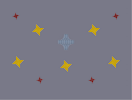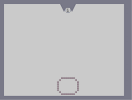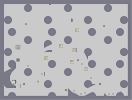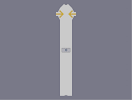Heaven 3D Rotating Triangle Sansui The Grim Baldur's Gate Paralyzed

Pages: (0)

### Sorry, I will not finish it.

My computer broke and we had to buy a new one, a pretty sucky one. When I load it, my pc freezes, so I'll have to wait until I buy another computer...

### ah - i thought it was from neon genesis

my tip here is - if you notice the wing things on the image - they are pretty symetrical - your ones arnt and they seem to be too fat. also i think you should have done the border smaller so that the wings can have the wide spread they need.
will be interesting to see the final result.

### i hope it looks better

when you finish it... the resemblance is not too good...